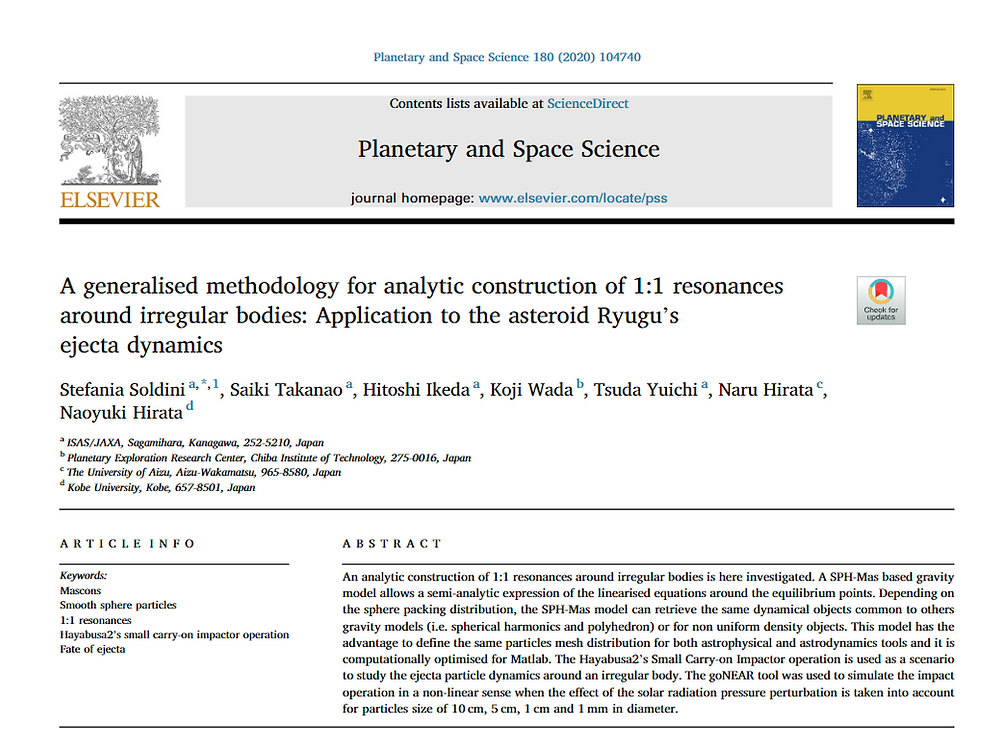Search
• RocketStefy

# Modelling rubble pile asteroids like spheres cluster (journal article)

Updated: Jun 12, 2020

I hope you will enjoy our article! (link to the article)In this article, we discuss about the effect of the internal structure of an asteroid on the dynamical objects. What are dynamical objects in Celestial Mechanics? they are elements that describe the qualitative motion of a particle around a celestial body, in our case, asteroids. We model the gravity starting from the classical Newton's Law of Universal gravitation and use a multi point mass gravity model (Mascons Gravity Model) to investigate the effect of sphere packing structures onto those objects.

The figure below shows the location of the equilibrium points (dynamical objects that can be seen in a rotating frame). We found out that the initial distribution of spheres (point masses) influences the number and locations of those equilibrium zones (a particle on this point will stay in a orbital period equal to the asteroid rotation as geostationary orbits for the Earth).

When using hydrocodes and sphere packing for simulating asteroid's impact event, be careful on the initial distribution of the sphere as you might start your impact simulations with initial conditions that are not preserving the gravity and dynamical properties of the asteroid! This would be an inconsistency in your simulation. We are interested in finding a common modeling method for both hydrocodes (astrophysics tools) and N-Body planetary simulations (astrodynamics tools).We also identified semi-analytic formula to compute periodic orbits around those equilibrium points in the linearised dynamics. Useful for initiating numerical continuation methods for finding periodic orbits in a non-linear sense. For the case of Ryugu, 15 families of periodics orbit exist around the six equilibrium points.For finding the periodic orbits in the figure above, we use as a reference the uniform density polyhedron gravity model as show in the figure below. Left: the Zero Velocity Curves that determine a qualitative boundary of motion related to a particle's energy). Right: the effective potential associated to the polyhedron gravity model.